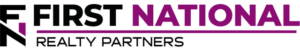# 5 Excel Formulas for Real Estate Investment Analysis

### Key Takeaways

• Prior to committing capital to any commercial real estate investment, it is important for investors to perform financial due diligence on the property.
• Financial due diligence involves the creation of a proforma and the analysis of the resulting cash flows.
• To complete a proforma, the most useful tool is Microsoft Excel, which has powerful analysis features that can be used to analyze private real estate cash flows.
• There are a nearly infinite number of Excel functions that can be used in financial analysis, but there are five that are particularly relevant to real estate investment: NPV, IRR/XIRR, PMT, DateDif, and Sum/Average.

Fundamentally, private commercial real estate analysis seeks to compare the cost of an asset to the cash flow that it produces. While the concept is relatively simple, the real estate analysis itself can be much more complicated in practice. There are numerous variables to consider, and an abundance of complex math to perform. For this reason, it is common to use a spreadsheet tool, such as Microsoft Excel, to assist with real estate analysis.

Excel is a powerful program that can perform a near-infinite number of calculations, but there can be a learning curve for those unfamiliar with the nomenclature and actions needed to get the most out of the tool. As it relates to private real estate analysis, there are five formulas that all investors and analysts should know to fully understand an investment’s risk/return profile.

In this article, we explain five Excel formulas for real estate analysis, including net present value, internal rate of return, payment, date difference, and sum and average.

## But First, It Starts With the Pro Forma

Every financial model starts with a pro forma, which is an estimate of the property’s income, expenses, and debt service for the entirety of the planned investment holding period.

Pro forma income is derived from tenant rents and ancillary fees. Rents are obtained from reviewing the property’s rent roll and/or individual leases. These documents provide key information about things like the lease start date, end date, rent amount, and rental escalations, if any. Separately, ancillary fees are obtained from a property’s historical operating statements.

Expenses are obtained from a combination of the property’s historical financial statements and the actual invoices for things like property taxes, insurance, maintenance, and property management. Gross income less operating expenses equals a key real estate metric known as Net Operating Income (NOI). This is a critical input to a property’s valuation calculation.

Next, a property’s debt service is calculated using the loan amount, interest rate, and amortization period. This value is subtracted from NOI to determine the cash available for distribution (sometimes called pre-tax cash flow), which is another key investment analysis metric. When complete, the pro forma may look something like this: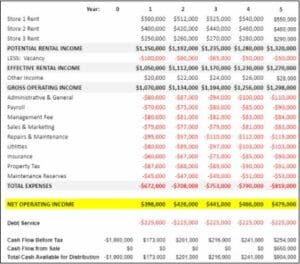To create the pro forma and analyze a property’s projected cash flows, there are five Excel functions that all investors should be aware of.

## Excel Formula #1: NPV – Net Present Value

The “NPV” function calculates the net present value of a series of cash flows by using a discount rate and a series of future cash flows. The “syntax” for the function is:

=NPV (rate, value 1, value 2,…)

Using the sample pro forma above, the NPV of the cash flows (assuming a 7% discount rate) can be calculated by replacing the variables in the above function with the actual cash flows as follows:

=NPV(7%, -1,000,000, \$173,000, \$201,000, \$216,000, \$241,000, \$904,000)

The result of this calculation is \$319,590. What does this mean? It means that there is a big difference between the discount rate of 7% and the internal rate of return (IRR), which is the next function that real estate investors should be familiar with.

## Excel Formula #2: IRR/XIRR – Internal Rate of Return

The Internal Rate of Return is the discount rate that sets the NPV of future cash flows equal to zero. The syntax used in the function is:

=IRR(values)

In other words, when the discount rate used in the NPV function is the same as the IRR, the result is \$0. Replacing the variables in the IRR function with the actual cash flows from the pro forma looks like this:

=IRR(-1,000,000, \$173,000, \$201,000, \$216,000, \$241,000, \$904,000)

The result of the calculation is 16.01%, which represents that annual rate of return on this series of cash flows. If this value is used as the discount rate in the NPV calculation, the result should be \$0 or close to \$0.

In private commercial real estate transactions, the return is calculated using a modified version of the IRR function known as XIRR. The key difference between the two is that IRR assumes regular intervals between periodic cash flows. XIRR uses the exact number of days between two cash flows. To calculate XIRR, an additional input is needed and that is the dates of each cash flow. These are shown in the table below:The resulting XIRR is 15.99%, which is only slightly different from 16.01%. However, this difference is magnified if the cash flows are more irregular and can have a major impact on the cash flow splits between a private equity sponsor and their investors.

## Excel Formula #3: PMT – Payment

Any real estate financial modeling exercise must include a calculation of the property’s loan payments. This is made possible by using the Excel “PMT” function. The required syntax is:

=PMT(rate, nper, pv)

Assume that the loan for the property is \$2.8M with an interest rate of 5%, and an amortization period of 240 months. To calculate the annual debt service, the variables in the function can be replaced with the actual numbers as follows:

=PMT(5%, 240/12, \$2,800,000)

The result is \$224,679 per year. The importance of this number is that it is subtracted from Net Operating Income to calculate the pre-tax cash flow for the property.

## Excel Formula #4: DateDif – Date Difference

To calculate XIRR and other precise return metrics, it is often necessary to calculate the number of days between two periods. This task can be easily completed by using the “DateDif” function. The required syntax is:

=DateDif(Start Date, End Date, Unit)

The last part of the function indicates the unit in which to return the difference. Common options are days (“d”), months (“m”), and years (“y”). To illustrate how this works, assume that a real estate investor was trying to determine the number of days between 01/01/20 and 03/25/20, which are the dates of quarterly cash distributions. The DateDif function can be used to calculate this as follows:

=DateDif(01/01/20,03/25/20,”d”)

## Excel Formula #5: Sum and Average

To calculate total or average cash flows for a given period of time, it is necessary to be familiar with the Sum and Average Excel functions. Respectively, their syntax is as follows:

=SUM(Value 1, Value 2…)

=Average(Value 1, Value 2…)

To illustrate how these functions are used in real estate analysis, calculate the sum and average of the following series of cash flows: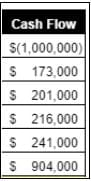The sum of this series is \$735,000 and the Average is \$122,500.

## Summary and Conclusions

In a typical private commercial real estate investment opportunity, transaction sponsors will create their own pro forma and perform their own financial analysis. These calculations are summarized in the investment’s offering materials. However, it is incumbent upon individual investors to perform their own analysis, using an Excel spreadsheet, to test the sponsors’ assumptions and to make sure they are comfortable with the risk/return profile of the real estate investment opportunity.

## Interested In Learning More?

First National Realty Partners is one of the country’s leading private equity commercial real estate investment firms. We leverage our decades of expertise and our available liquidity to find world-class, multi-tenanted assets below intrinsic value. In doing so, we seek to create superior long-term, risk-adjusted returns for our investors while creating strong economic assets for the communities we invest in.

Get instant access to all of our current and past commercial real estate deals.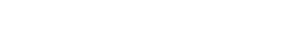## Subscribe Now

Get the latest news on real estate

1031 Exchange

Acquisitions

CRE Basics

## Get More From FNRP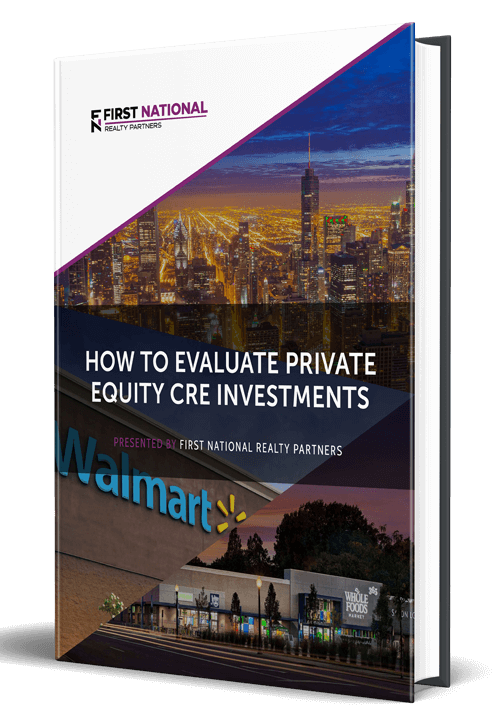## How to Evaluate Private Equity CRE Investments## How to Complete a 1031 Exchange with a Private Equity Sponsor